# 游戏AI之模糊逻辑

if(condition)
then dosomething...


## 模糊变量

#### 隶属函数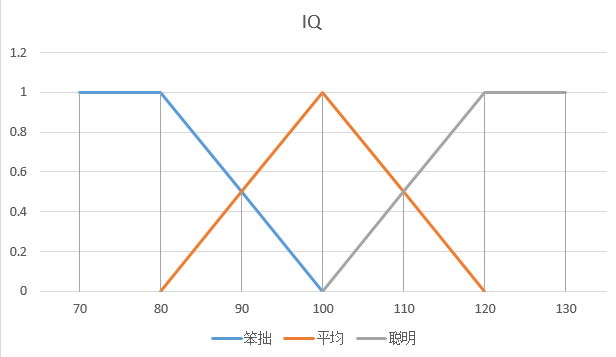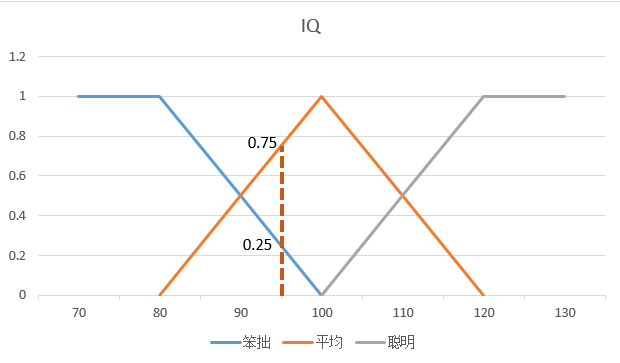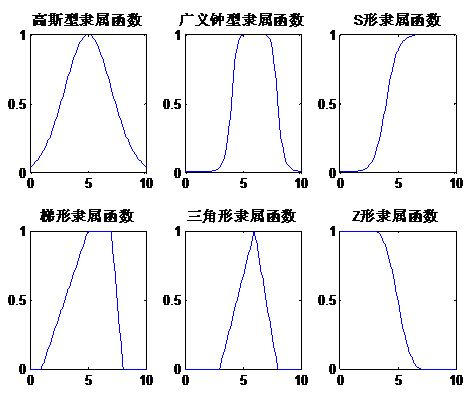#### 限制词

• “非常”：$$F_{Very}(x) = F(x)^2$$
• “有点”：$$F_{Fairly}(x) = \sqrt{F(x)}$$

“非常笨拙”的隶属度 = “笨拙”的隶属度的平方 = 0.25^2 = 0.0625。

## 模糊集合

#### 模糊集合运算符

• OR或 : $$F_{1}(x)$$ OR $$F_{2}(y)$$ = max{$$F_{1}(x)$$, $$F_{2}(y)$$}

• AND与 : $$F_{1}(x)$$ AND $$F_{2}(y)$$ = min{$$F_{1}(x)$$, $$F_{2}(y)$$}

• NOT反 : $$F(x)^{`} = 1-F(x)$$

## 模糊规则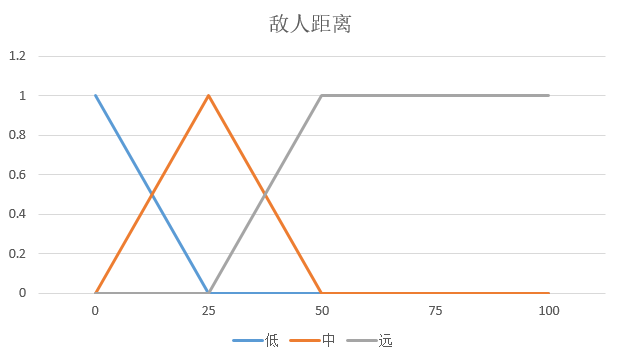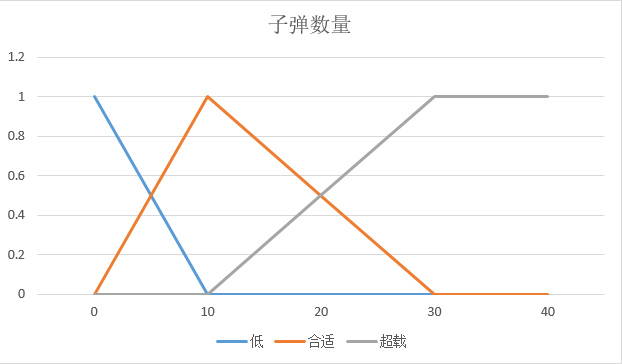• 模糊变量1：敌人距离 = {近,中,远}
• 模糊变量2：子弹数量 = {低,合适,超载}

• 规则1:if(子弹数量低 AND 敌人距离远)then 不期望攻击
• 规则2:if(子弹数量低 AND 敌人距离中)then 不期望攻击
• 规则3:if(子弹数量低 AND 敌人距离近)then 期望攻击
• 规则4:if(子弹数量合适 AND 敌人距离近)then 非常期望攻击

• 规则1中：不期望攻击的隶属度 = “子弹数量低” AND “敌人距离远” 的隶属度
• 规则2中：不期望攻击的隶属度 = “子弹数量低” AND “敌人距离中” 的隶属度
• 规则3中：期望攻击的隶属度 = “子弹数量低” AND “敌人距离近” 的隶属度
• 规则4中：非常期望攻击的隶属度 = “子弹数量合适” AND “敌人距离近” 的隶属度

## 模糊推理

• 敌人距离近的隶属度 = 0.5
• 敌人距离中的隶属度 = 0.5
• 敌人距离远的隶属度 = 0
• 子弹数量低的隶属度 = 0.25
• 子弹数量合适的隶属度 = 0.75
• 子弹数量超载的隶属度 = 0

• 规则1：不期望攻击的隶属度 = 0
• 规则2：不期望攻击的隶属度 = 0.25
• 规则3：期望攻击的隶属度 = 0.25
• 规则4：非常期望攻击的隶属度 = 0.5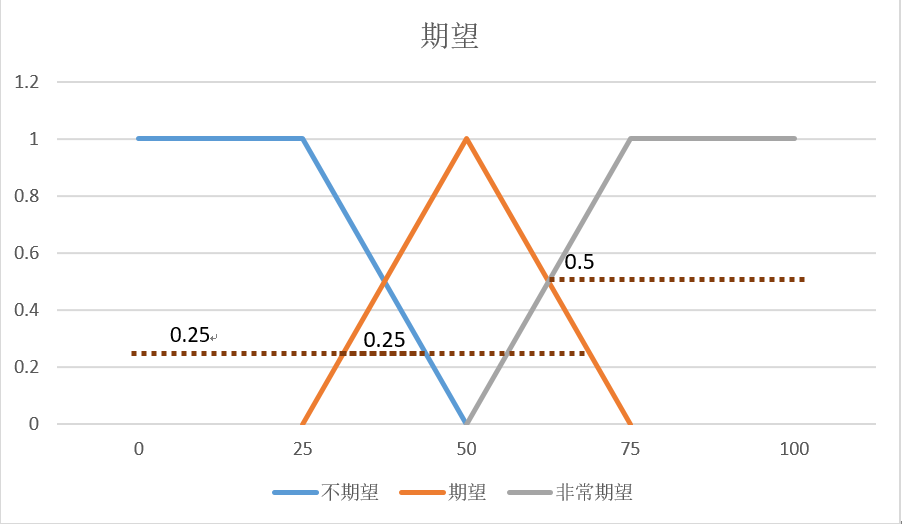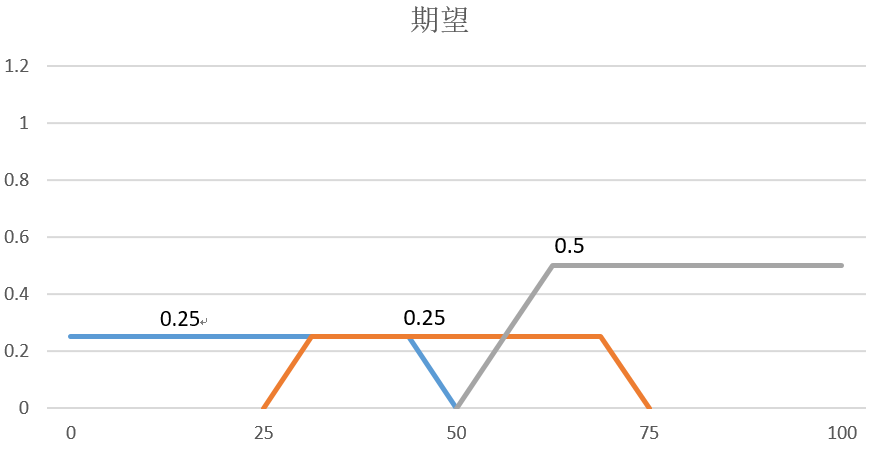## 去模糊化

#### 最大值平均

OutPut = $$\frac{0.25*21.875+0.25*50+0.5*81.25}{0.25+0.25+0.5}$$ = 58.59375

#### 中心法

N越大，其结果越准确。

$$OutPut = \frac{\sum\limits_{i=1}^N{(x_{i}\sum\limits_{j=1}^M F_j(x_{i}))}}{\sum\limits_{i=1}^N{\sum\limits_{j=1}^M F_j(x_{i})}}$$

M为被剪裁后的形状（函数）的数量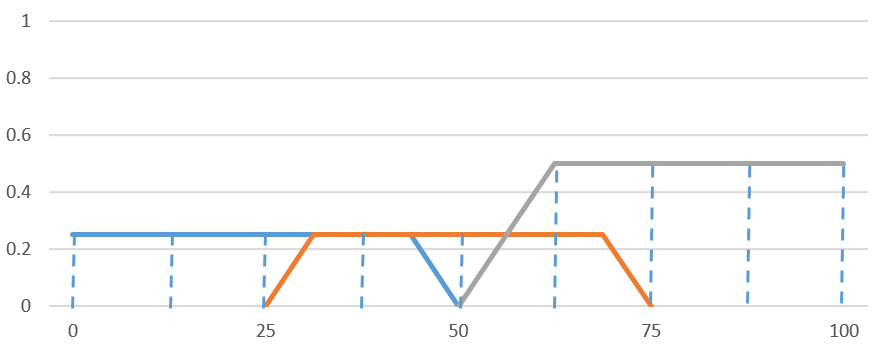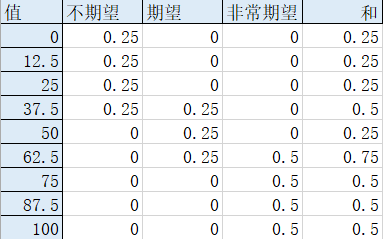OutPut = $$\frac{0*0.25+12.5*0.25+25*0.25+37.5*0.25+37.5*0.5+50*0.25+62.5* 0.75+75*0.5+87.5*0.5+100*0.5}{0.25+0.25+0.25+0.5+0.25+0.75+0.75+0.5+0.5}$$
= 57.03125

## 库博方法

• if("聪明"和“长”)then 非常期望
• if("聪明"和“中”)then 非常期望
• if("聪明"和“短”)then 期望
• if("平均"和“长”)then 非常期望
• if("平均"和“中”)then 期望
• if("平均"和“短”)then 期望
• if("笨拙"和“长”)then 期望
• if("笨拙"和“中”)then 期望
• if("笨拙"和“短”)then 不期望

• if("聪明")then 非常期望
• if("平均")then 期望
• if("笨拙")then 不期望
• if(“长”)then 非常期望
• if(“中”)then 期望
• if(“短”)then 不期望

## 结语

posted @ 2019-02-06 00:09  KillerAery  阅读(3938)  评论(2编辑  收藏  举报